Courses

# RD Sharma Solutions -Ex-8.1, Introduction To Algebra, Class 6, Maths Class 6 Notes | EduRev

## RD Sharma Solutions for Class 6 Mathematics

Created by: Abhishek Kapoor

## Class 6 : RD Sharma Solutions -Ex-8.1, Introduction To Algebra, Class 6, Maths Class 6 Notes | EduRev

The document RD Sharma Solutions -Ex-8.1, Introduction To Algebra, Class 6, Maths Class 6 Notes | EduRev is a part of the Class 6 Course RD Sharma Solutions for Class 6 Mathematics.
All you need of Class 6 at this link: Class 6

Question 1: Write the following using numbers, literals and signs of basic operations. State what each letter represents:

(i) The diameter of a circle is twice its radius.

(ii) The area of a rectangle is the product of its length and breadth.

(iii) The selling price equals the sum of the cost price and profit.

(iv) The total amount equals the sum of the principal and the interest.

(v) The perimeter of a rectange is two times the sum of its length and breadth.

(vi) The perimeter of a square is four times its side.

Solution:

(i) Let r and d be the radius and diameter of the circle, respectively.

Therefore, d = 2r

(ii) Let l and b be the length and breadth of the rectangle. respectively.

Therefore, area of rectangle = lb

(iii) Let s, c and p be the selling price, cost price and profit, respectively.

Therefore, s = c + p

(iv) Let T, p and i be the total amount, principal and interest, respectively.

Therefore, T = p + i

(v) Let land b be the length and breadth of the rectangle, respectively.

Therefore, perimeter of rectangle = 2(l + b)

(vi) Let a be the side of the square.

Therefore, permiter of the square = 4a

Question 2: Write the following using numbers, literals and signs of basic operations:

(i) The sum of 6 and x.

(ii) 3 more than a number y.

(iii) One- third of a number x.

(iv) One- half of the sum of number x and y.

(v) Number y less than a number 7.

(vi) 7 taken away from x.

(vii) 2 less than the quotient of x by y

(viii) 4 times x taken away from one-thirs of y.

(ix) Quotient of x by 3 is multiplies by y.

(i) The sum of 6 and x is 6 + x.

(ii) 3 more than a number y means y + 3.

(iii) One-third of a number x is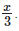.

(iv) One-half of the sum of numbers x and y is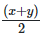.

(v) Number y less than a number 7 means 7 - y.

(vi) 7 taken away from x means x - 7.

(vii) 2 less than the quotient of x by y is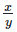−2.

(viii) 4 times x taken away from one-third of y is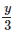−4x.

(ix) Quotient of x by 3 is multiplied by y means: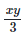Question 3: Think of a number. Multiply it by 5. Add 5 to the result. Subtract y from this result. What is the result?

Answer: Let the number be x.

On multiplying the number by 5. we get: 5x

Further, adding 6 to 5x. we get: 5x + 6

Finally, on subtracting y from 5x + 6,

we get: 5x + 6 – y

Question 4: The number of rooms on the ground floor of a building is 12 less than the twice of the numbers of rooms on the first floor. If the first floor has x rooms, how many rooms does the ground floor has?

Answer: Let the number of rooms on the ground floor be y.

It is given that the number of rooms on the first floor is x; therefore, we have:

y =2 X x - 12

= 2x - 12

Thus, the number of rooms on the ground floor is 2x - 12.

Question 5: Binny spends Rs. A daily and saves Rs. B per week. What is her income for 2 weeks?

Answer: It is given that Binny spends Rs. a in one day.

Money spent by him in one week = 7 X a = 7a

It is further given that he saves Rs. b in one week; therefor we have:

Total income in one week = Total expenditure in one week + Total saving in one week

= 7a + b

Therefore, Binny's total income in 2 weeks = 2 X (7a + b)

= Rs. (14a + 2b)

Question 6: Rahul score 80 marks in English and x marks in Hindi. What is his total scores in the two subjects?

Answer: Marks obtained in English = 80

Marks obtained in Hindi = x

Total marks obtained = 80 + x

Question 7: Rohit covers x centimeters in one step. How much distance does he covers in y steps?

Answer: It is given that Rohit covers x cm in one step.

Therefore, distance covered by him in y steps = x X y = x y cm

Question 8: One apple weighs 75 grams and one orange weighs 40 grams. Determine the weigh of x apples an y oranges.

Answer: Weight of an apple = 75 grams

Wright of an orange = 40 grams

Weight of x apples = 75 X x = 75x grams

Weight of y oranges = 40 X y = 40y grams

Total weight of x apples and y oranges = (75x + 40y) grams

Question 9: One pencil costs Rs. 2 and one fountain pen costs Rs. 15. What is the cost of x pernicls and y fountain pens?

Answer: Cost of one pencil = Rs. 2

Cost of x pencils = Rs. 2x

Cost of one fountain pen = Rs. 15

Cost of y fountain pens = Rs. 15y

Total cost of x pencils and y fountain pens = Rs. (2x + 15y)

103 docs

,

,

,

,

,

,

,

,

,

,

,

,

,

,

,

,

,

,

,

,

,

,

,

,

,

,

,

,

,

,

;